Linear Static

NAS 101: Linear Static and Normal Modes Analysis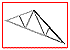Linear Static Analysis of a Simply-Supported Truss: Define a set of material properties for the previous exercise using the beam library and perform static analysis under 3 separate loading conditions.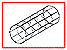Rigid Element Analysis with RBAR: Create a tube model with a 'rigid' end and apply a torque load for linear static analysis.Rigid Element Analysis with RBE2 and CONM2: Create a tube model with a 'rigid' end, define mass concentrations and apply a gravitational load for linear static analysis.

NAS103: MSC/NASTRAN Nonlinear Analysis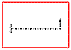Geometric Linear Analysis of a Cantilever Beam: Perform linear analysis on a cantilever beam under four increasing loads, create an accurate deformation plot of the model and a plot of the load factor vs. displacement.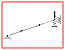Linear Buckling Load Analysis (without spring): Demonstrate the use of linear buckling analysis.

NAS120: Linear Static and Normal Nodes Analysis Using MSC/NASTRAN and MSC/PATRAN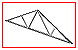Linear Static Analysis of a Simply-Supported Truss: Create a finite element truss with segments in tension or compression connected by smooth pins.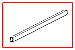Linear Static Analysis of a Cantilever Beam (SI Units): Create and apply a pure bending load to a hollow, rectangular beam.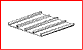Linear Static Analysis of a Simply-Supported Stiffened Plate: Create a stiffened plate, prepare a MSC/NASTRAN input file for a Linear Static analysis and visualize results.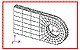Linear Static Analysis of a Solid Lug: Create a geometrical cantilever lug, define nonuniform loads using Fields, prepare file for MSC/NASTRAN input and visualize analysis results.Restarting a Linear Static Analysis of a Simply-Supported Stiffened Plate: Restart previous Stiffened Plate for new load conditions.Linear Static - Rigid Element Analysis with RBE2 and CONM2: Run previous lessons using linear static analysis.Linear Static Analysis of a Cantilever Beam Using Beam Library (SI Units): Create and apply a pure bending load to a hollow, rectangular beam using beam library.